# Methods and Differences in Current Regeneration for PWM Driving of Brushed Motors

2022.12.07

In this article, we describe methods of current regeneration for PWM driving of brushed motors, as well as differences between them.

## Methods of Current Regeneration in PWM Driving of Brushed Motors

In PWM driving of a brushed motor, methods for current regeneration in off duty intervals can be broadly classified into two types. One type is methods that are equivalent to short-circuiting the two terminals of the brushed motor. The other type is methods in which the two terminals of the brushed motor are equivalently connected to the power supply with the polarities reversed relative to the on-duty intervals. Below, these methods are explained in detail.

## Regeneration Methods in Which the Two Motor Terminals are Equivalently Shorted

Fig. 1 shows the equivalent circuits for when current is supplied in PWM driving (on-duty intervals), and for the method of current regeneration in which the two motor terminals are equivalently shorted. Turned-off transistors are omitted.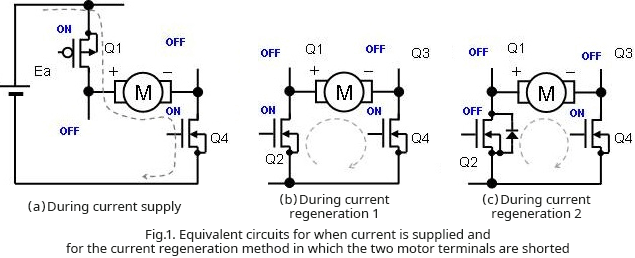In (a), during current supply, Q1 and Q4 are turned on and the motor is connected to the power supply. There are two methods of equivalently short-circuiting the two terminals of the motor. In (b), which shows the circuit for current regeneration method 1, starting from the state in (a), Q1 is turned off to cut off the connection to the power supply, and by turning on Q2 and leaving Q4 on, the motor terminals are short-circuited. In the current regeneration method 2 shown in (c), Q1 is similarly turned off, but Q2 remains off, and Q4 is also left on, and so the motor current circulates via the parasitic diode of Q2.
?
Fig. 2 shows PWM operation waveforms when the method of current regeneration by shorting the two motor terminals is used. Because the equivalent circuits for (b) and (c) are basically the same, the waveforms are also the same. The changes between peaks in the current waveform are shown somewhat larger than the actual changes to aid understanding.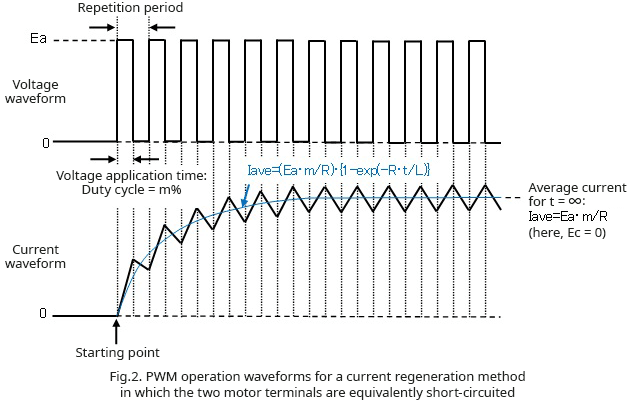The average motor current value Iave for the case in which time t is ∞, that is, in the steady state, is as follows when the voltage generated by the motor (Ec) is set to zero.

Iave=Ea･m/R  where Ea: motor power supply voltage, m: on-duty ratio, R: motor equivalent resistance

Hence a voltage equal to the on-duty ratio m multiplied by the motor power supply voltage Ea is applied to the motor.

## Regeneration Methods in Which the Two Motor Terminals are Equivalently Connected to the Power Supply with the Reversed Polarity to On-Duty Intervals

Next, Fig. 3 shows equivalent circuits for the other type of regeneration method, in which the two terminals of the motor are equivalently connected to the power supply with the polarity opposite that used for on-duty intervals. This method also has two variations.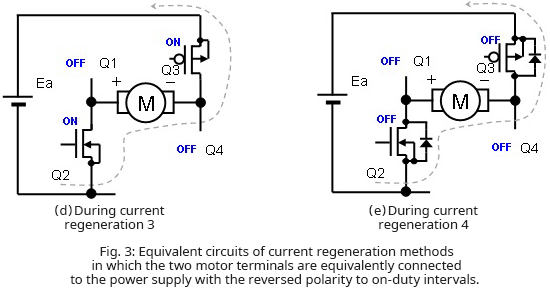In current regeneration method 3 in (d), Q1 and Q4 are turned off, Q2 and Q3 are on, and the polarity of the power supply applied to the motor is the opposite of the polarity during on-duty intervals. In this circuit, a backward current flows depending on the duty ratio. In current regeneration method 4 shown in (e), all transistors are turned off, but current is regenerated through the parasitic diodes of Q2 and Q4, equivalently reversing the polarity of the on-duty intervals and the power supply to the motor. Because the connection is effected by diodes, a backward current does not flow in this circuit.

Fig. 4 shows PWM operation waveforms for a circuit in which, as in (d), the power supply with equivalently reversed polarity relative to on-duty intervals is connected to the two motor terminals and current regeneration occurs, so that a current flows in the backward direction in the circuit.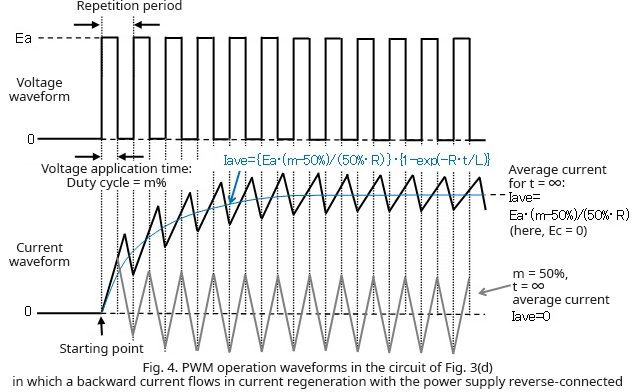In the circuit (d), because a backward current flows when the regeneration current falls to zero or lower, at an on-duty of 50% the average motor current becomes zero. At on duties from 100% to 50%, the average current flows in a certain direction; from 50% to 0%, the average current flows in the direction opposite the direction when the on-duty is above 50%. The equivalent voltage applied to the motor is as follows.

Equivalent voltage applied to motor＝Ea・(m－50%)/50%  where Ea: motor power supply voltage, m: on-duty ratio

Fig. 5 shows PWM operation waveforms for a circuit in which, as in (e), current regeneration occurs upon reversing the power supply connection, but a backward current does not flow.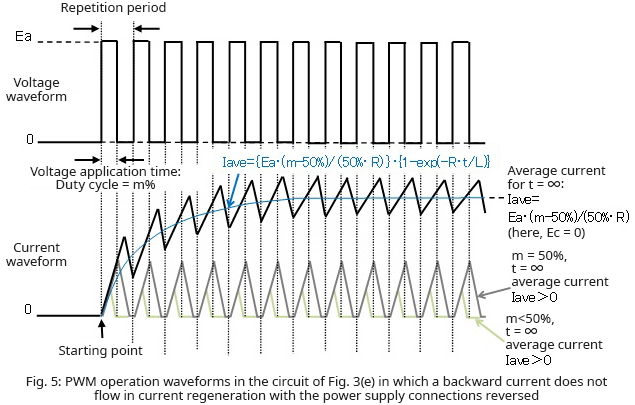In the circuit of (e), the current does not flow in the direction opposite the current direction during on-duty intervals, so that the average current does not go to zero even when the on-duty ratio is 50%; as the on-duty ratio is reduced from 50%, the average current decreases, reaching zero at a ratio of 0%. The equivalent voltage applied to the motor when the minimum current peak value is greater than zero due to the transient current waveform is as follows.

Equivalent voltage applied to motor＝Ea・(m－50%)/50%  where Ea: motor power supply voltage, m: on-duty ratio

In all of these circuits, the actually applied equivalent voltage is somewhat different from the value obtained by the calculations shown above, owing to the difference in on resistances of the high side and low side output transistors (MOSFETs) and the forward voltages of the parasitic diodes.

## Summary

In the regeneration methods of Fig. 1-(b) and (c) in which the two motor terminals are equivalently short-circuited, attenuation of the regeneration current is gradual. On the other hand, in the current regeneration methods of Fig. 3-(d) and (e) in which the connections of the two motor terminals to the power supply are reversed in polarity relative to the on-duty intervals, because a voltage acting to pass current in the opposite direction acts on the coils, the regeneration current attenuation is rapid.

In regeneration methods that equivalently short-circuit the two motor terminals, the voltage applied to the motor in PWM operation is more or less the voltage equal to the power supply voltage times the on-duty ratio. On the other hand, in regeneration methods in which the connection of the two motor terminals to the power supply is equivalently reversed in polarity relative to the on-duty intervals, a voltage approximately equal to the power supply voltage × (on-duty ratio – 50%)/50% is applied.

Among the regeneration methods in which the connections of the two motor terminals to the power supply are equivalently reversed in polarity relative to the on-duty intervals, in circuit (d) in which output transistors are turned on and connections are reversed, a backward current flows, and so a backward current flows when on-duty ratio is lower than 50%. In the case of the circuit (e) in which output transistors are all turned off and the reverse connection is due to parasitic diodes, on the other hand, a backward current does not flow, and so a current due to the on-duty ratio flows in the positive direction even when the on-duty ratio is lower than 50%.

Regeneration method Regeneration current attenuation Fig. 1-(b), (c)Two motor terminals equivalently shorted Fig. 3-(d), (e)Power supply connections to two motor terminals equivalently reversed Gradual Rapid Ea・m Ea・(m－50%)/50% Ea・m/R Ea・(m－50%)/(50%・R) *In (d), current flows in the backward direction, so that for m＝50% the current is zero, and when m＜50%, a negative current flows. *In (e), a current does not flow in the backward direction, so that even when m?50%, only a positive current flows.

※Ea: motor power supply voltage, m: on-duty ratio, R: motor equivalent resistance